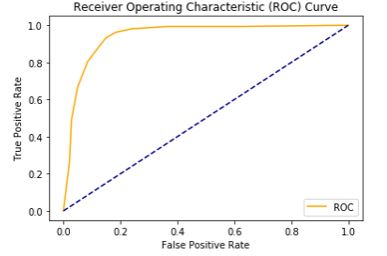# How to plot ROC Curve using Sklearn library in Python

In this tutorial, we will learn an interesting thing that is how to plot the roc curve using the most useful library Scikit-learn in Python.  This tutorial is a machine learning-based approach where we use the sklearn module to visualize ROC curve.

## What is Scikit-learn library?

• Scikit-learn was previously known as scikits .learn.
• It is an open-source library which consists of various classification, regression and clustering algorithms to simplify tasks.
• It is mainly used for numerical and predictive analysis by the help of the Python language.

### What is the ROC curve?

• receiver operating characteristic curve, commonly known as the ROC curve.
• It is an identification of the binary classifier system and discrimination threshold is varied because of the change in parameters of the binary classifier system.
• The ROC curve was first developed and implemented during World War -II by the electrical and radar engineers.
• It has one more name that is the relative operating characteristic curve. Therefore has the diagnostic ability.

#### What are TPR and FPR?

• TPR stands for True Positive Rate and FPR stands for False Positive Rate.
• Both the parameters are the defining factors for the ROC curve and are known as operating characteristics.
• True Positive Rate as the name suggests itself stands for ‘real’ sensitivity and It’s opposite False Positive Rate stands for ‘pseudo’ sensitivity.

For further reading and understanding, kindly look into the following link below.

Python program:

Step 1:
Import all the important libraries and functions that are required to understand the ROC curve, for instance, numpy and pandas.

```import numpy as np
import pandas as pd
import matplotlib.pyplot as plt
import seaborn as sns
from sklearn.datasets import make_classification
from sklearn.neighbors import KNeighborsClassifier
from sklearn.ensemble import RandomForestClassifier
from sklearn.model_selection import train_test_split
from sklearn.metrics import roc_curve
```

Step 2:
Define the function and place the components.

```def plot_roc_cur(fper, tper):
plt.plot(fper, tper, color='orange', label='ROC')
plt.plot([0, 1], [0, 1], color='darkblue', linestyle='--')
plt.xlabel('False Positive Rate')
plt.ylabel('True Positive Rate')
plt.legend()
plt.show()```

NOTE: Proper indentation and syntax should be used.

Step 3:
Now use the classification and model selection to scrutinize and random division of data.

```data_X, cls_lab = make_classification(n_samples=1100, n_classes=2, weights=[1,1], random_state=1)
train_X, test_X, train_y, test_y = train_test_split(data_X, cls_lab, test_size=0.3, random_state=1)```

Step 4:
Now use any algorithm to fit, that is learning the data.  However, I have used RandomForestClassifier.

```model = RandomForestClassifier()
model.fit(trainX, trainy)```

Output:

```RandomForestClassifier(bootstrap=True, class_weight=None, criterion='gini',
max_depth=None, max_features='auto', max_leaf_nodes=None,
min_impurity_decrease=0.0, min_impurity_split=None,
min_samples_leaf=1, min_samples_split=2,
min_weight_fraction_leaf=0.0, n_estimators=10, n_jobs=None,
oob_score=False, random_state=None, verbose=0,
warm_start=False)

```

Step 5:
Now plot the ROC curve, the output can be viewed on the link provided below.

```probs = model.predict_proba(testX)
probs = probs[:, 1]
fper, tper, thresholds = roc_curve(testy, probs)
plot_roc_curve(fper, tper)```

Output:

The output of our program will looks like you can see in the figure below:1.Akshat jain says: